# CBSE Physics Practical Class 11

CBSE Physics Practical Class 11 consists of various interesting experiments. Students are evaluated on these practicals and experiments at the end of the examinations. The marks of the Class 11 Physics practicals are added when the final score of students is prepared. Therefore, students must pay attention to Physics Practical Class 11 diligently to perform better in the final exam. CBSE provides a well-structured syllabus for the CBSE Practical Class 11 Physics examination. The Physics Lab Manual Class 11 contains all the experiments and activities which students have to perform in the laboratory.

CBSE Class 11 Physics Practical is provided here to help students prepare for the annual exams. The practical exam carries a total of 30 marks. Two experiments, one from each section, carry 8 marks, with a practical record (experiment and activities) for 2 marks and viva on experiments and activities carrying 5 marks. Along with the projects and experiments, students must also prepare for the viva questions on the experiments with the help of Physics Lab Manual Class 11. Students should practise graphs and diagrams as they are essential parts of the Physics Practical CBSE Class 11 exam. To download the CBSE Syllabus for Class 11 Physics Practicals, click on the link below.

## CBSE Class 11 Physics Practical Syllabus PDF 2023-24

### CBSE Syllabus for Class 11 Physics Practical Evaluation Scheme

Below you will find the evaluation scheme for Class 11 Physics Practical.

 Topics Marks Two experiments one from each section 7+7 Practical record (experiment and activities) 5 One activity from any section 3 Investigatory Project 3 Viva on experiments, activities and project 5 Total 30

### CBSE Class 11 Physics Practical Syllabus 2023-24

Below you will find a list of detailed information on experiments, activities and projects in PDF format for students of Class 11.

 Sl. No Practical Syllabus 1 Major Skills in Physics Practical Work 2 Experiments 1 to 5 3 Experiments 6 & 7 4 Experiment 8 5 Experiments 10 6 Experiments 11 to 13 7 Experiments 14 to 17 8 Activities 1 to 5 9 Activities 6 to 13 10 Projects 1 to 8 11 Project 9 12 Projects 10 & 11 13 Demonstrations

### Physics Practical Class 11 CBSE Syllabus: List of Experiments

Below you will find a list of section-wise experiments for Class 11 Physics.

### CBSE Class 11 Physics Experiments

Section A
1. To measure diameter of a small spherical/cylindrical body and to measure internal diameter and depth of a given beaker/calorimeter using Vernier Callipers and hence find its volume
2. To measure diameter of a given wire and thickness of a given sheet using screw gauge.
3. To determine volume of an irregular lamina using screw gauge
4. To determine radius of curvature of a given spherical surface by a spherometer.
5. To determine the mass of two different objects using a beam balance.
6. To find the weight of a given body using parallelogram law of vectors.
7. Using a simple pendulum, plot its L-T2 graph and use it to find the effective length of second’s pendulum.
8. To study variation of time period of a simple pendulum of a given length by taking bobs of same size but different masses and interpret the result.
9. To study the relationship between force of limiting friction and normal reaction and to find the co- efficient of friction between a block and a horizontal surface
10. To find the downward force, along an inclined plane, acting on a roller due to gravitational pull of the earth and study its relationship with the angle of inclination θ by plotting graph between force and Sinθ.
Section B
1. To determine Young’s modulus of elasticity of the material of a given wire.
2. To find the force constant of a helical spring by plotting a graph between load and extension.
3. To study the variation in volume with pressure for a sample of air at constant temperature by plotting graphs between P and V, and between P and 1/V.
4. To determine the surface tension of water by capillary rise method.
6. To study the relationship between the temperature of a hot body and time by plotting a cooling curve.
7. To determine specific heat capacity of a given solid by method of mixtures.
8. To study the relation between frequency and length of a given wire under constant tension using sonometer.
9. To study the relation between the length of a given wire and tension for constant frequency using sonometer.
10. To find the speed of sound in air at room temperature using a resonance tube by two resonance positions.

## CBSE Class 11 Physics Practical Syllabus: List of  Activities

Below you will find a list of CBSE Class 11 Physics Activities for students.

Section A

1. To make a paper scale of given least count, e.g., 0.2cm, 0.5 cm.

2. To determine mass of a given body using a metre scale by principle of moments.

3. To plot a graph for a given set of data, with proper choice of scales and error bars.

4. To measure the force of limiting friction for rolling of a roller on a horizontal plane.

5. To study the variation in range of a projectile with angle of projection.

6. To study the conservation of energy of a ball rolling down on an inclined plane (using a double inclined plane).

7. To study dissipation of energy of a simple pendulum by plotting a graph between square of amplitude and time.

Section B

1. To observe change of state and plot a cooling curve for molten wax.

2. To observe and explain the effect of heating on a bi-metallic strip.

3. To note the change in level of liquid in a container on heating and interpret the observations.

4. To study the effect of detergent on surface tension of water by observing capillary rise.

5. To study the factors affecting the rate of loss of heat of a liquid.

6. To study the effect of load on depression of a suitably clamped metre scale loaded at (i) its end (ii) in the middle.

7. To observe the decrease in pressure with increase in velocity of a fluid.

## Physics Lab Manual Class 11

Students can have a look at the details of a few experiments mentioned in the Physics Lab Manual Class 11.

EXPERIMENT-1
AIM:APPARATUS AND MATERIAL REQUIRED: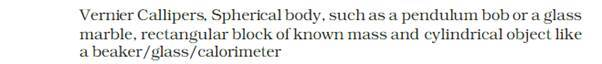DESCRIPTION OF THE MEASURING DEVICE: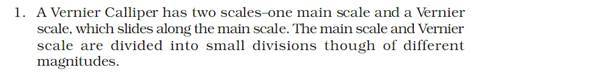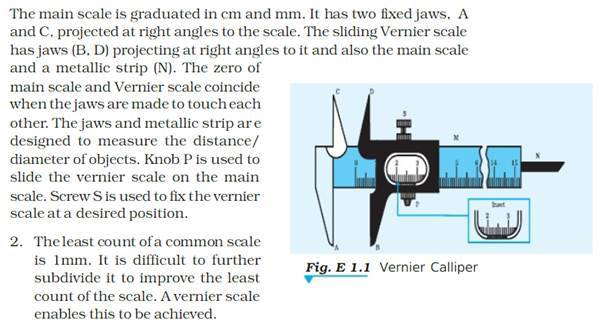PRINCIPLE: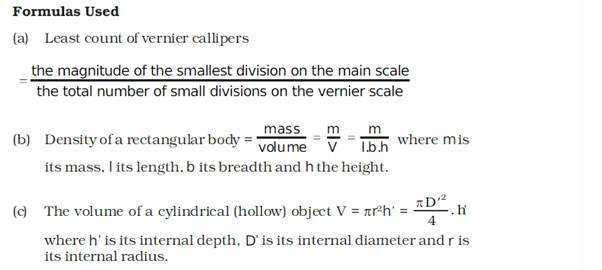PROCEDURE: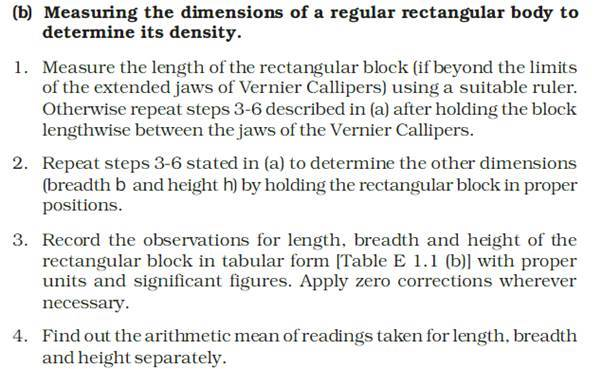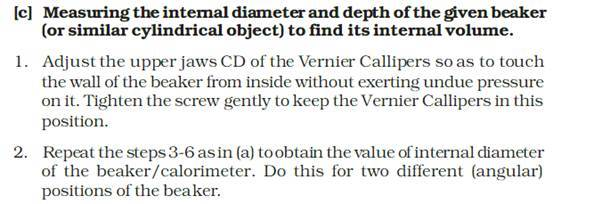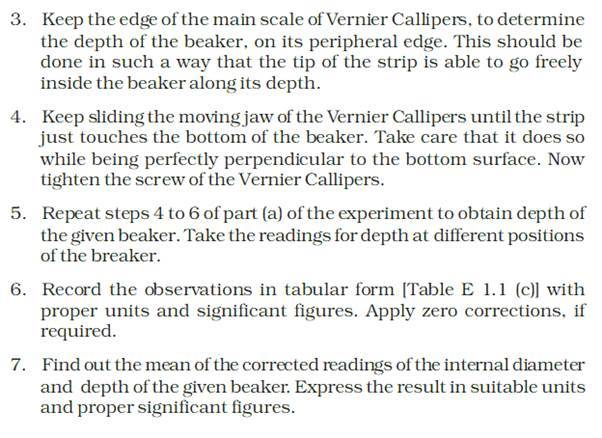OBSERVATIONS: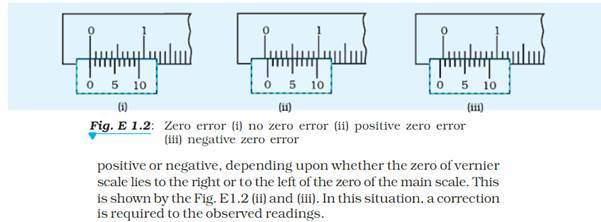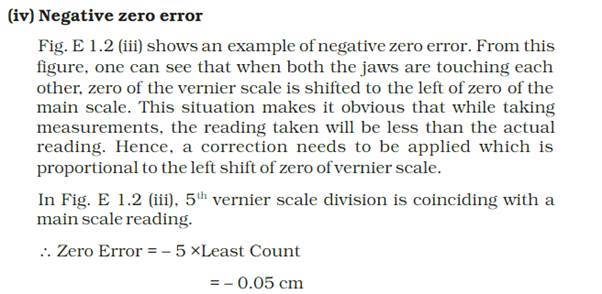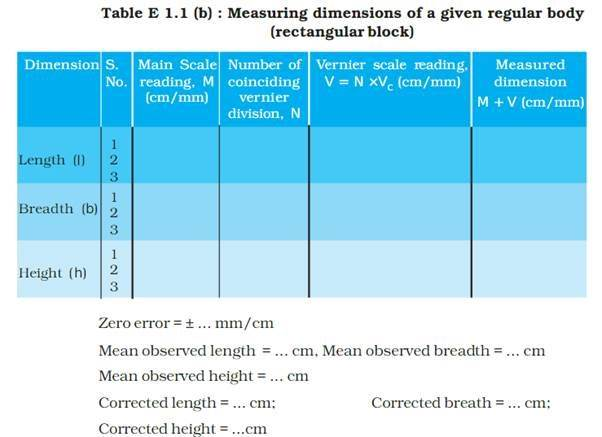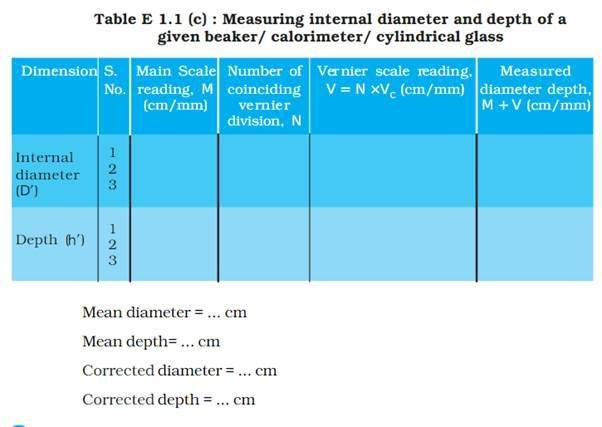CALCULATION:RESULT:PRECAUTIONS: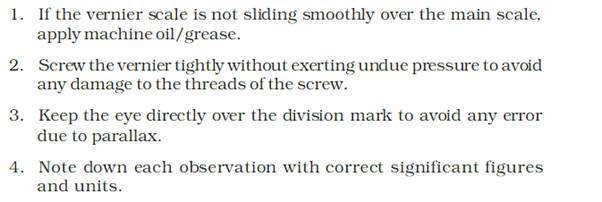SOURCES OF ERROR: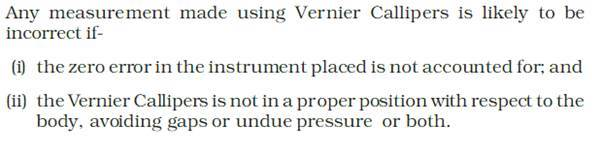EXPERIMENT 2
AIM:APPARATUS AND MATERIAL REQUIRED: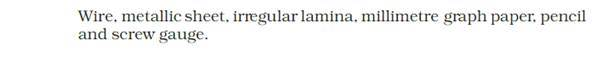DESCRIPTION OF APPARATUS: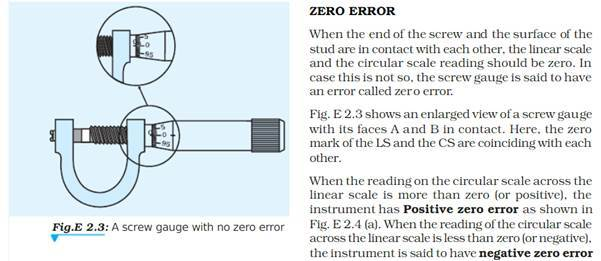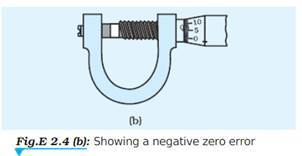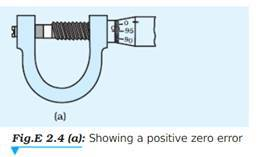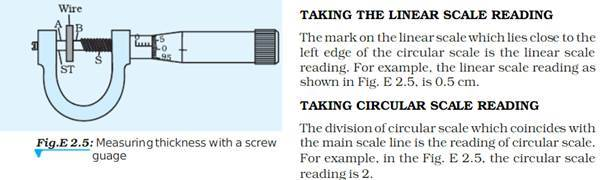PRINCIPLE: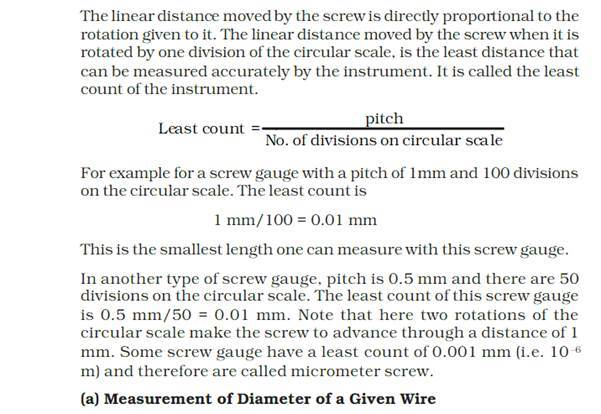PROCEDURE: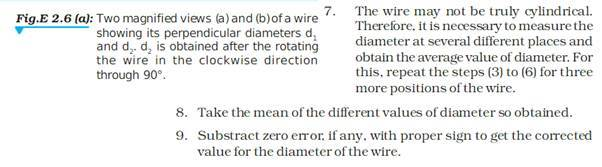OBSERVATIONS AND CALCULATION:RESULT:PRECAUTIONS: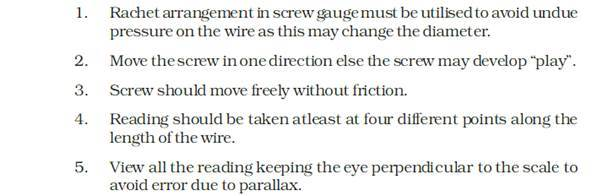SOURCES OF ERROR: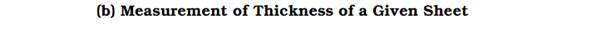PROCEDURE:OBSERVATIONS AND CALCULATION: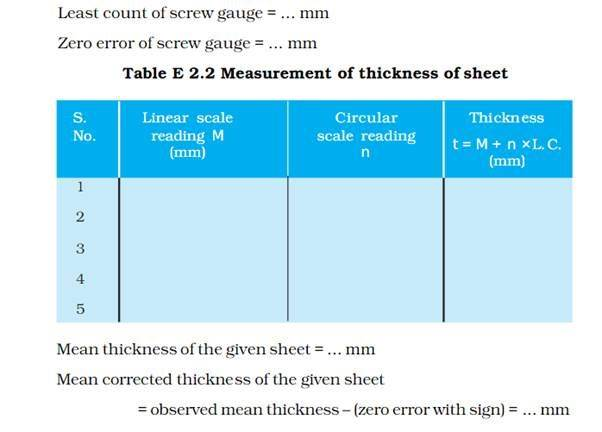RESULT:PROCEDURE: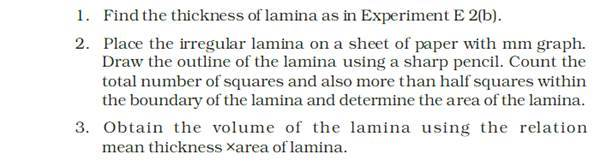OBSERVATIONS AND CALCULATION: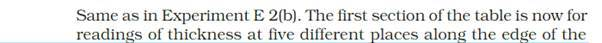RESULT:Stay tuned to BYJU’S to get the latest notifications on CBSE exams along with the CBSE syllabus, sample papers, marking scheme and more.